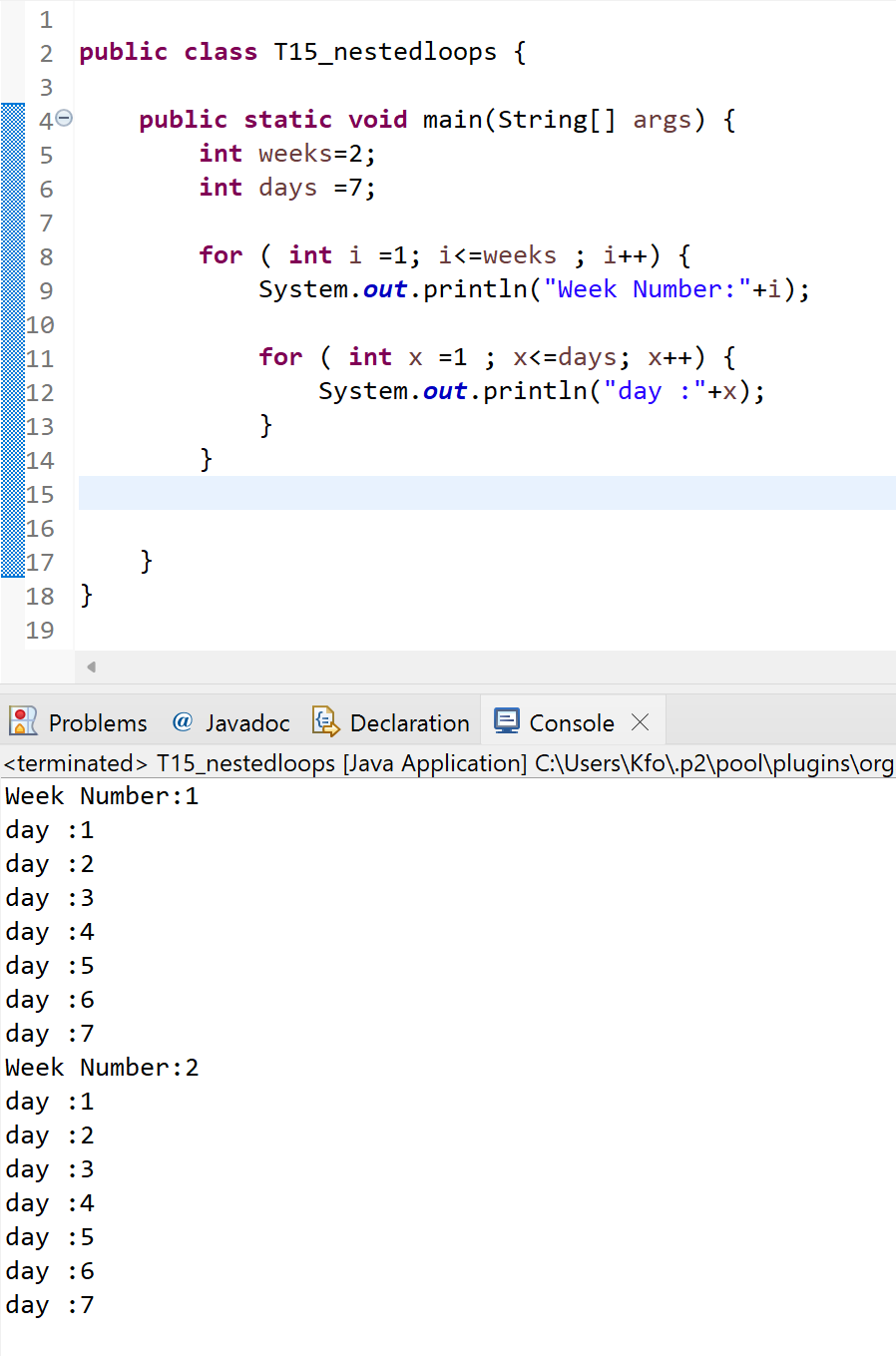•A.razaq

# T15-Nested loops

in this tutorial we will learn how to use nested loops

and nested loops are basically loop statement inside another loop statement

Syntax :

```
// outer loop
for (int i = 1; i <= 5; ++i) {
// our code here
// inside loop
for(int j = 1; j <=2; ++j) {
// our code here
}
}```

lets take an example

```int row = 10;
for (int i = 0; i<=row; i++) {

for ( int x=0; x<=i; x++) {
System.out.print(x+" ");
}

System.out.println("");
}```

the output for this nested loop will be :Note : a common mistake is using the same variable from the first loop into the second loop , every loop must begins with a new variable

in the example below first loop variable is ( i ) and 2nd loop variable is (x)

another example :

```	int weeks=2;
int days =7;

for ( int i =1; i<=weeks ; i++) {
System.out.println("Week Number:"+i);

for ( int x =1 ; x<=days; x++) {
System.out.println("day :"+x);
}
}```

and the output for this one will be :